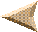These tables are formatted as follows. Each table contains cross-coupled generalized structure coefficients at a single structural degree s. Each row of each table contains the cross-coupled structure coefficients for a pair of multiplets. Cross-coupled structure coefficients can be either of even or odd degree depending on the differenece in harmonic degree of the two coupling multiplets and the two mode types.
The first 6 columns specify the n, type, and l of the pair of coupled multiplets and the final 2s+1 columns specify the degree s structure coefficients.
n1 s1/t1 l1 n2 s2/t2 l2 c1 c2 c3 ...... c(2s+1)
The structure coefficients are normalized relative to fully normalized complex spherical harmonics (e.g, Edmonds, 1960) which are commonly used in normal mode studies (e.g., Woodhouse, 1980). For example, for degree 2 there are 5 coefficients: c20, Re c21, Im c21, Re c22, Im c22. For degree 4 there are 9 coefficients, etc. The units of the coefficients are micro-Hz.
A discussion of the generalized structure coefficients can be found by clicking the following: 1, 2, 3. A discussion of normalization is on the second page.If you have questions, comments or suggestions, email Mike Ritzwoller at ritzwoller@phys-geophys.colorado.eduReturn to Normal Mode Seismology Research Page.Return to Geophysics Group Home Page.